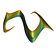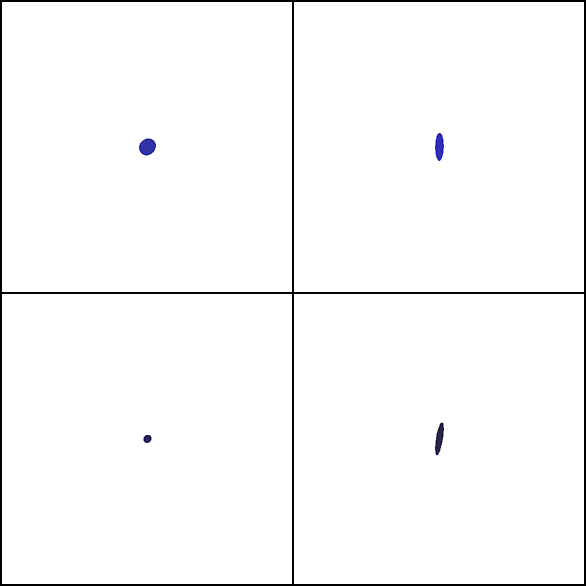Previous: The stable manifold# Each ring is a levelset of the cost function on the manifold of locally optimal solutions

Looking down the x-axis
an (x,y,z)-projection
Looking down the x-axis
an (x,y,w)-projectionLooking down the z-axis
an (x,z,w)-projection
Looking down the z-axis
a (y,z,w)-projection

Previous: The stable manifold